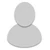## FANDOM

3,745 Pages

• If a character, strong enough to lift a building, throws a punch at mach 50, how much force would that yield?

• Pretty sure it might gonna be 9-A.

• How do you figure that?

• Honestly i don't know if it was gonna be correct to give reasons about it to answer or not, i might be wrong about it.

• I'll calc the punch. KE = 1/2M*V^2

Average human hand mass = 310 grams = 0.31 KG

V = mach 50 = 17150 meters per second

KE = 1/2(0.31)*(17150)^2 = 45,588,987.5 J. which is 9-A, Small Building level

If I did this wrong, please tell me.

I'm not doing the building part because buildings can vary. a lot.

• Drag-O-Drawgon wrote:
I'll calc the punch. KE = 1/2M*V^2

Average human hand mass = 310 grams = 0.31 KG

V = mach 50 = 17150 meters per second

KE = 1/2(0.31)*(17150)^2 = 45,588,987.5 J. which is 9-A, Small Building level

If I did this wrong, please tell me.

I'm not doing the building part because buildings can vary. a lot.

I know this is VERY late, but punches are calculated with the entire mass of an arm, which is approximately 6.5% of the total body weight of a person.

•